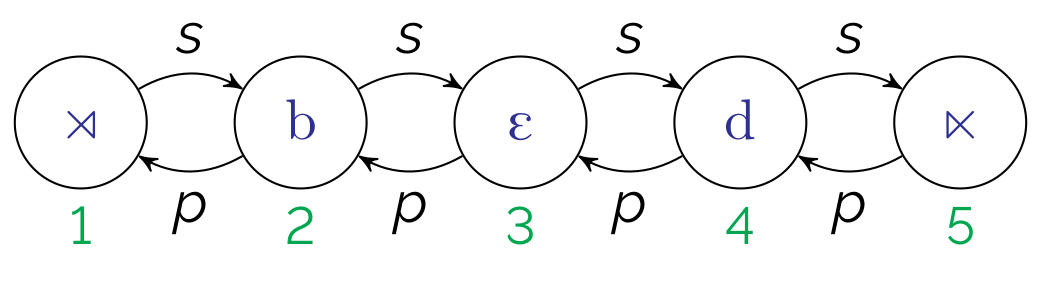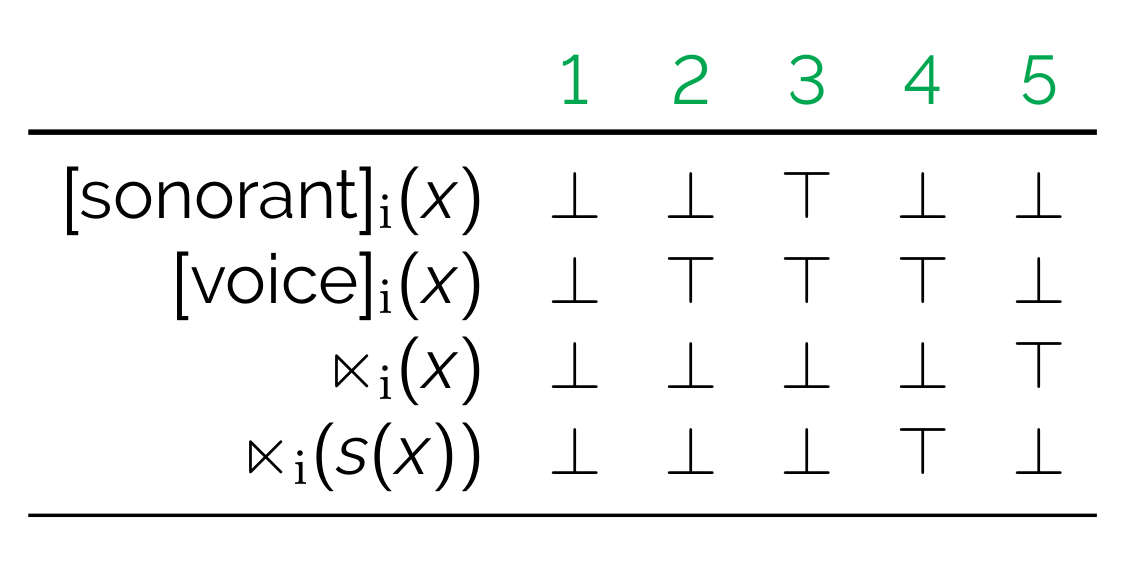# String models

• A string model explicitly defines all of the elements in the string with:
• indices (elements in the structure)
• order functions $$p$$ and $$s$$
• properties of the indices• We'll assume a set of (privative) featural properties• Note:
• $$\top$$ refers to true, $$\bot$$ false
• $$\rtimes$$ and $$\ltimes$$ represent boundaries (i.e., the equivalent of #)